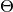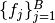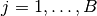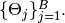# Model¶

The `Model` type is designed to store the set of all model nodes, including parameter setas denoted in the Mamba Gibbs sampling scheme. In particular, it stores `Dependent` type objects in its `nodes` dictionary field. Valid models are ones whose nodes form directed acyclic graphs (DAGs). Sampling functionsare saved as `Sampler` objects in the vector of field `samplers`. Vector elementscorrespond to sampling blocks## Declaration¶

`type Model`

## Fields¶

• `nodes::Dict{Symbol, Any}` : all input, logical, and stochastic model nodes.
• `samplers::Vector{Sampler}` : sampling functions for updating blocks of stochastic nodes.
• `states::Vector{ModelState}` : states of chains at the end of an MCMC run in a possible series of runs, where `ModelState` has fields `value::Vector{Float64}` and `tune::Vector{Any}` to store the last values of sampled nodes and block-sampler tuning parameters, respectively.
• `iter::Int` : current MCMC draw from the target distribution.
• `burnin::Int` : number of initial draws to discard as a burn-in sequence to allow for convergence.
• `hasinputs::Bool` : whether values have been assigned to input nodes.
• `hasinits::Bool` : whether initial values have been assigned to stochastic nodes.

## Constructor¶

`Model`(; iter::Integer=0, burnin::Integer=0, samplers::Vector{Sampler}=Sampler[], nodes...)

Construct a `Model` object that defines a model for MCMC simulation.

Arguments

• `iter` : current iteration of the MCMC simulation.
• `burnin` : number of initial draws to be discarded as a burn-in sequence to allow for convergence.
• `samplers` : block-specific sampling functions.
• `nodes...` : arbitrary number of user-specified arguments defining logical and stochastic nodes in the model. Argument values must be `Logical` or `Stochastic` type objects. Their names in the model will be taken from the argument names.

Value

Returns a `Model` type object.

Example

See the Model Specification section of the tutorial.

## MCMC Engine¶

`mcmc`(m::Model, inputs::Dict{Symbol}, inits::Vector{Dict{Symbol, Any}}, iters::Integer; burnin::Integer=0, thin::Integer=1, chains::Integer=1, verbose::Bool=true)
`mcmc`(mc::ModelChains, iters::Integer; verbose::Bool=true)

Simulate MCMC draws for a specified model.

Arguments

• `m` : specified model.
• `mc` : chains from a previous call to `mcmc` for which to simulate additional draws.
• `inputs` : values for input model nodes. Dictionary keys and values should be given for each input node.
• `inits` : dictionaries that contain initial values for stochastic model nodes. Dictionary keys and values should be given for each stochastic node. Consecutive runs of the simulator will iterate through the vector’s dictionary elements.
• `iters` : number of draws to generate for each simulation run.
• `burnin` : numer of initial draws to discard as a burn-in sequence to allow for convergence.
• `thin` : step-size between draws to output.
• `chains` : number of simulation runs to perform.
• `verbose` : whether to print sampler progress at the console.

Value

A `ModelChains` type object of simulated draws.

Example

See the MCMC Simulation section of the tutorial.

## Indexing¶

`getindex`(m::Model, nodekey::Symbol)

Returns a model node identified by its symbol. The syntax `m[nodekey]` is converted to `getindex(m, nodekey)`.

Arguments

• `m` : model containing the node to get.
• `nodekey` : node to get.

Value

The specified node.
`keys`(m::Model)
`keys`(m::Model, ntype::Symbol, at...)

Extract the symbols (keys) for all existing nodes or for nodes of a specified type.

Arguments

• `m` : model containing the nodes of interest.
• `ntype` : type of nodes to return. Options are
• `:all` : all input, logical, and stochastic model nodes.
• `:assigned` : nodes that have been assigned values.
• `:block` : stochastic nodes being updated by the sampling block(s) `at::Integer=0` (default: all blocks).
• `:dependent` : logical and stochastic (dependent) nodes in topologically sorted order.
• `:independent` or `:input` : input (independent) nodes.
• `:logical` : logical nodes.
• `:monitor` : stochastic nodes being monitored in MCMC sampler output.
• `:output` : stochastic nodes upon which no other stochastic nodes depend.
• `:source` : nodes upon which the node `at::Symbol` or vector of nodes `at::Vector{Symbol}` depends.
• `:stochastic` : stochastic nodes.
• `:target` : topologically sorted nodes that depend on the sampling block(s) `at::Integer=0` (default: all blocks), node `at::Symbol`, or vector of nodes `at::Vector{Symbol}`.
• `at...` : additional positional arguments to be passed to the `ntype` options, as described above.

Value

A vector of node symbols.

## Display¶

`draw`(m::Model; filename::AbstractString="")

Draw a GraphViz DOT-formatted graph representation of model nodes and their relationships.

Arguments

• `m` : model for which to construct a graph.
• `filename` : external file to which to save the resulting graph, or an empty string to draw to standard output (default). If a supplied external file name does not include a dot (`.`), the file extension `.dot` will be appended automatically.

Value

The model drawn to an external file or standard output. Stochastic, logical, and input nodes will be represented by ellipses, diamonds, and rectangles, respectively. Nodes that are unmonitored in MCMC simulations will be gray-colored.

Example

See the Directed Acyclic Graphs section of the tutorial.
`graph`(m::Model)

Construct a graph representation of model nodes and their relationships.

Arguments

• `m` : model for which to construct a graph.

Value

Returns a `ModelGraph` type object with field `graph` containing a `DiGraph` representation of indices, as defined in the LightGraphs package, to a vector of node symbols in field `keys`.
`graph2dot`(m::Model)

Draw a GraphViz DOT-formatted graph representation of model nodes and their relationships.

Arguments

• `m` : model for which to construct a graph.

Value

A character string representation of the graph suitable for in-line processing. Stochastic, logical, and input nodes will be represented by ellipses, diamonds, and rectangles, respectively. Nodes that are unmonitored in MCMC simulations will be gray-colored.

Example

See the Directed Acyclic Graphs section of the tutorial.
`show`(m::Model)

Write a text representation of the model, nodes, and attributes to the current output stream.

`showall`(m::Model)

Write a verbose text representation of the model, nodes, and attributes to the current output stream.

## Initialization¶

`setinits!`(m::Model, inits::Dict{Symbol, Any})

Set the initial values of stochastic model nodes.

Arguments

• `m` : model with nodes to be initialized.
• `inits` : initial values for stochastic model nodes. Dictionary keys and values should be given for each stochastic node.

Value

Returns the model with stochastic nodes initialized and the `iter` field set equal to 0.

Example

See the Development and Testing section of the tutorial.
`setinputs!`(m::Model, inputs::Dict{Symbol, Any})

Set the values of input model nodes.

Arguments

• `m` : model with input nodes to be assigned.
• `inputs` : values for input model nodes. Dictionary keys and values should be given for each input node.

Value

Returns the model with values assigned to input nodes.

Example

See the Development and Testing section of the tutorial.
`setsamplers!`(m::Model, samplers::Vector{T<:Sampler})

Set the block-samplers for stochastic model nodes.

Arguments

• `m` : model with stochastic nodes to be sampled.
• `samplers` : block-specific samplers.

Values:

Returns the model updated with the block-samplers.

Example

See the Model Specification and MCMC Simulation sections of the tutorial.

## Parameter Block Operations¶

`gettune`(m::Model, block::Integer=0)

Get block-sampler tuning parameters.

Arguments

• `m` : model with block-samplers.
• `block` : block for which to get the tuning parameters (default: all blocks).

Value

A `Vector{Any}` of all block-specific tuning parameters if `block=0`, and turning parameters for the specified block otherwise.
`gradlogpdf`(m::Model, block::Integer=0, transform::Bool=false; dtype::Symbol=:forward)
`gradlogpdf`(m::Model, x::AbstractVector{T<:Real}, block::Integer=0, transform::Bool=false; dtype::Symbol=:forward)
`gradlogpdf!`(m::Model, x::AbstractVector{T<:Real}, block::Integer=0, transform::Bool=false; dtype::Symbol=:forward)

Compute the gradient of log-densities for stochastic nodes.

Arguments

• `m` : model containing the stochastic nodes for which to compute the gradient.
• `block` : sampling block of stochastic nodes for which to compute the gradient (default: all stochastic nodes).
• `x` : value (possibly different than the current one) at which to compute the gradient.
• `transform` : whether to compute the gradient of block parameters on the link–transformed scale.
• `dtype` : type of differentiation for gradient calculations. Options are
• `:central` : central differencing.
• `:forward` : forward differencing.

Value

The resulting gradient vector. Method `gradlogpdf!()` additionally updates model `m` with supplied values `x`.

Note

Numerical approximation of derivatives by central and forward differencing is performed with the Calculus package .
`logpdf`(m::Model, block::Integer=0, transform::Bool=false)
`logpdf`(m::Model, nodekeys::Vector{Symbol}, transform::Bool=false)
`logpdf`(m::Model, x::AbstractArray{T<:Real}, block::Integer=0, transform::Bool=false)
`logpdf!`(m::Model, x::AbstractArray{T<:Real}, block::Integer=0, transform::Bool=false)

Compute the sum of log-densities for stochastic nodes.

Arguments

• `m` : model containing the stochastic nodes for which to evaluate log-densities.
• `block` : sampling block of stochastic nodes over which to sum densities (default: all stochastic nodes).
• `nodekeys` : nodes over which to sum densities.
• `x` : value (possibly different than the current one) at which to evaluate densities.
• `transform` : whether to evaluate evaluate log-densities of block parameters on the link–transformed scale.

Value

The resulting numeric value of summed log-densities. Method `logpdf!()` additionally updates model `m` with supplied values `x`.
`sample!`(m::Model, block::Integer=0)

Generate one MCMC sample of values for a specified model.

Argument:

• `m` : model specification.
• `block` : block for which to sample values (default: all blocks).

Value

Returns the model updated with the MCMC sample and, in the case of `block=0`, the `iter` field incremented by 1.

Example

See the Development and Testing section of the tutorial.
`unlist`(m::Model, block::Integer=0, transform::Bool=false)
`unlist`(m::Model, nodekeys::Vector{Symbol}, transform::Bool=false)
`relist`(m::Model, x::AbstractArray{T<:Real}, block::Integer=0, transform::Bool=false)
`relist`(m::Model, x::AbstractArray{T<:Real}, nodekeys::Vector{Symbol}, transform::Bool=false)
`relist!`(m::Model, x::AbstractArray{T<:Real}, block::Integer=0, transform::Bool=false)
`relist!`(m::Model, x::AbstractArray{T<:Real}, nodekey::Symbol, transform::Bool=false)

Convert (unlist) sets of logical and/or stochastic node values to vectors, or reverse (relist) the process.

Arguments

• `m` : model containing nodes to be unlisted or relisted.
• `block` : sampling block of nodes to be listed (default: all blocks).
• `nodekey/nodekeys` : node(s) to be listed.
• `x` : values to re-list.
• `transform` : whether to apply a link transformation in the conversion.

Value

The `unlist` methods return vectors of concatenated node values, `relist` return dictionaries of symbol keys and values for the specified nodes, and `relist!` return their model argument with values copied to the nodes.
`update!`(m::Model, block::Integer=0)
`update!`(m::Model, nodekeys::Vector{Symbol})

Update values of logical and stochastic model node according to their relationship with others in a model.

Arguments

• `m` : mode with nodes to be updated.
• `block` : sampling block of nodes to be updated (default: all blocks).
• `nodekeys` : nodes to be updated in the given order.

Value

Returns the model with updated nodes.